• 根号n段归并排序算法时间复杂度分析过程： 1.合并 根号n向下取整 段子数组使用的是自底向上两两归并的策略 2.根号n段归并排序算法时间复杂度的数学推导
• ## 归并排序时间复杂度分析

万次阅读 多人点赞 2019-03-19 18:04:57
归并排序时间复杂度分析归并排序工作原理时间复杂度计算如何插入一段漂亮的代码片KaTeX数学公式 归并排序 归并排序也叫（Merge sort）。 工作原理 将给定的数组一份为二 对两部分数组再使用归并排序使其有序 最后再...


归并排序时间复杂度分析
归并排序工作原理时间复杂度计算

归并排序
归并排序也叫（Merge sort）。
工作原理
将给定的数组一份为二对两部分数组再使用归并排序使其有序最后再将两部分数组合并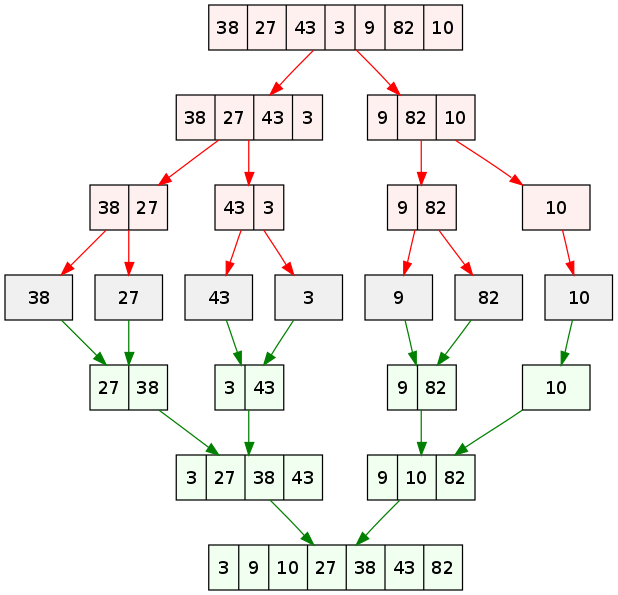时间复杂度计算
1、首先可知

f

(

n

)

=

2

f

(

n

2

)

+

n

f(n)=2f(\frac{n}{2})+n \space\space\space\space\space\space\space\space\space

其中：

f

(

n

)

表

示

对

n

个

数

进

行

归

并

排

序

f(n)表示对n个数进行归并排序

2

f

(

n

2

)

表

示

将

n

个

数

分

成

两

部

分

分

别

进

行

归

并

排

序

2f(\frac{n}{2})表示将n个数分成两部分分别进行归并排序

n

表

示

对

两

个

子

过

程

结

束

之

后

合

并

的

过

程

n表示对两个子过程结束之后合并的过程

2、推导

f

(

n

2

)

=

2

f

(

n

4

)

+

n

2

当

n

=

n

2

时

f(\frac{n}{2})=2f(\frac{n}{4})+\frac{n}{2} \space\space\space\space\space\space\space\space\space 当n=\frac{n}{2}时

f

(

n

4

)

=

2

f

(

n

8

)

+

n

4

当

n

=

n

4

时

f(\frac{n}{4})=2f(\frac{n}{8})+\frac{n}{4} \space\space\space\space\space\space\space\space\space 当n=\frac{n}{4}时

…

…

…… \space\space\space\space\space\space\space\space\space

f

(

n

2

m

−

1

)

=

2

f

(

n

2

m

)

+

n

2

m

−

1

当

n

=

n

2

m

−

1

时

f(\frac{n}{2^{m-1}})=2f(\frac{n}{2^m})+\frac{n}{2^{m-1}} \space\space\space\space\space\space\space\space\space 当n=\frac{n}{2^{m-1}}时

3、由此可得：

f

(

n

)

=

2

f

(

n

2

)

+

n

f(n)=2f(\frac{n}{2})+n

=

2

×

(

2

f

(

n

4

)

+

n

2

)

+

n

= 2\times\bigg(2f(\frac{n}{4})+\frac{n}{2}\bigg)+n

=

2

2

f

(

n

2

2

)

+

2

n

=2^2f(\frac{n}{2^2})+2n

=

2

2

×

(

2

f

(

n

8

)

+

n

4

)

+

2

n

=2^2\times\bigg(2f(\frac{n}{8})+\frac{n}{4}\bigg)+2n

=

2

3

f

(

n

2

3

)

+

3

n

=2^3f(\frac{n}{2^3})+3n

…

…

……

=

2

m

f

(

n

2

m

)

+

m

n

=2^mf(\frac{n}{2^m})+mn

当 m 足够大时(仅剩一个数字时)，可使得

n

2

m

=

1

\frac{n}{2^m}=1

求出

m

=

l

o

g

2

n

m=log_2n

代

入

f

(

n

)

=

2

m

f

(

n

2

m

)

+

m

n

中

可

得

代入 \space\space f(n)=2^mf(\frac{n}{2^m})+mn \space\space 中可得

f

(

n

)

=

2

(

l

o

g

2

n

)

f

(

1

)

+

n

⋅

l

o

g

2

n

f(n)=2^{(log_2n)}f(1)+n\cdot log_2n

其

中

f

(

1

)

=

0

其中\space\space f(1)=0

所

以

最

终

f

(

n

)

=

n

⋅

l

o

g

2

n

所以最终\space\space f(n)=n\cdot log_2n


展开全文分治算法 算法
• ## 归并排序时间复杂度过程分析

万次阅读 多人点赞 2019-07-08 20:54:43
归并排序方法就是把一组n个数的序列，折半分为两个序列，然后再将这两个序列再分，一直分下去，直到分为n个长度为1的...归并排序时间=分解时间+子序列排好序时间+合并时间 无论每个序列有多少数都是折中分解，...
https://blog.csdn.net/liangjiabao5555/article/details/89670082
归并排序方法就是把一组n个数的序列，折半分为两个序列，然后再将这两个序列再分，一直分下去，直到分为n个长度为1的序列。然后两两按大小归并。如此反复，直到最后形成包含n个数的一个数组。
归并排序总时间=分解时间+子序列排好序时间+合并时间
无论每个序列有多少数都是折中分解，所以分解时间是个常数，可以忽略不计。
则：归并排序总时间=子序列排好序时间+合并时间

如果假设一个序列有n个数的排序时间为T(n)，T(n)是一个关于n的函数，随着n的变化而变化。
那么我们将n个数的序列，分为两个(n/2)的序列。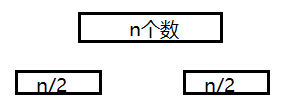那么T(n)=2*T(n/2)+合并时间
由于合并时，两个子序列已经组内排好序了，那我们将两个排好序的序列组合成一个大的有序序列，只需要一个if循环即可。if循环中有n个数需要比较，所以时间复杂度为n。
那么T(n)=2*T(n/2)+n

我们再将两个n/2个序列再分成4个(n/4)的序列。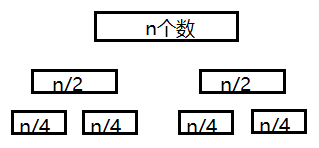一个（n/2）序列排序时间=两个(n/4)的序列排序时间+两个(n/4)的序列的合并为一个（n/2）的序列时间
T(n/2)=2*T(n/4)+n/2
将T(n/2)带入到T(n)中，T(n)=2*(2*T(n/4)+n/2)+n，
通过化简T(n)=4*T(n/4)+2n

我们再将4个n/4个序列再分成8个(n/8)的序列。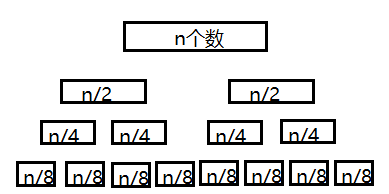T(n/4)=2*T(n/8)+n/4
将T(n/4)带入到黄色公式中，T(n)=4*(2*T(n/8)+n/4)+2n
通过化简T(n)=8*T(n/8)+3n
······

这样分下去，前面我们已经说过了，分为n个序列，每个序列里只有一个数为止。
前面我们假设的一个序列有n个数的排序时间为T(n)，现在每个序列只有一个数，所以不需要进行组内排序，时间复杂度为0。T(1)=0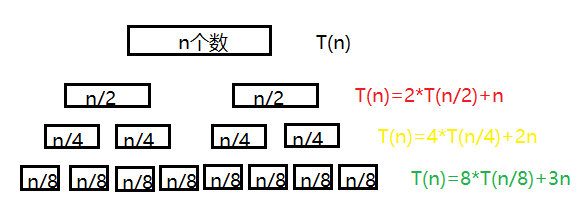大家有没有找到规律，右边式子中n前面的系数随着层数的增加而增加，第一层公式中没有n，则系数为0，第二层n的系数为1，第三层为2，第四层为3。那么规律就出来了，n前面的系数为层数减1。
那这个图有没有很熟悉，就像一个二叉树一样，通过二叉树的知识点我们可以知道，一个n个结点的二叉树层数为(log2n)+1。
那么n前面的系数为层数减1。
(log2n)+1-1=log2n
那log2n就是最底层n的系数。

那么我们最后一层是不是可以这样表示
T(n)=n*T(1)+(log2n)*n
T(1)=0，那么T(n)=(log2n)*n
所以归并排序的时间复杂度为nlog2n
展开全文• 归并排序算法 设置归并排序所化时间为 T(N)T(N)T(N)，其中 NNN 为输入数据长度 ...4、归并排序好的左边和右边元素，时间复杂度 θ(N)\theta(N)θ(N) 设置归并排序所化时间为 T(N)T(N)T(N)，其中 NNN 为输入
归并排序算法
设归并排序所花时间为

T

(

N

)

T(N)

，其中

N

N

为输入数据长度
1、当 n == 1 时，返回，时间复杂度

1

1

2、排序好左边

N

2

\frac{N}{2}

个元素，时间复杂度

T

(

N

/

2

)

T(N/2)

3、排序好右边

N

2

\frac{N}{2}

个元素，时间复杂度

T

(

N

/

2

)

T(N/2)

4、归并排序好的左边和右边元素，时间复杂度

θ

(

N

)

\theta(N)

根据上述算法有

T

(

N

)

=

{

1

N

=

1

2

T

(

N

/

2

)

+

θ

(

N

)

N

>

1

T(N)=\left\{\begin{aligned} & 1 & N = 1 \\ & 2T(N/2) + \theta(N) & N > 1 \\ \end{aligned}\right.

其中

θ

\theta

表示阶，

θ

(

N

)

\theta(N)

表示一阶，可以使用

c

N

cN

表示，其中

c

c

为常数。
递归树
使用递归树对公式进行分解如下图所示：最终可以分解为一个高度为

lg

⁡

N

\lg N

，每一层之和为

c

N

cN

的二叉递归树，

T

(

N

)

T(N)

即为树上每个节点之和，所以：

T

(

N

)

=

c

N

lg

⁡

N

=

θ

(

N

lg

⁡

N

)

+

θ

(

N

)

T(N) = cN\lg N = \theta(N\lg N) + \theta(N)

所以归并排序的时间复杂度为

θ

(

N

lg

⁡

N

)

\theta(N\lg N)

。
展开全文算法 线性代数
• 现在我们就借助归并排序来看看，如何用递归树，来分析递归代码的时间复杂度归并排序每次会将数据规模一分为二。我们把归并排序画成递归树，就是下面这个样子： 因为每次分解都是一分为二，所以代价很低，我们把...
归并排序算法你还记得吧？它的递归实现代码非常简洁。现在我们就借助归并排序来看看，如何用递归树，来分析递归代码的时间复杂度。
归并排序每次会将数据规模一分为二。我们把归并排序画成递归树，就是下面这个样子：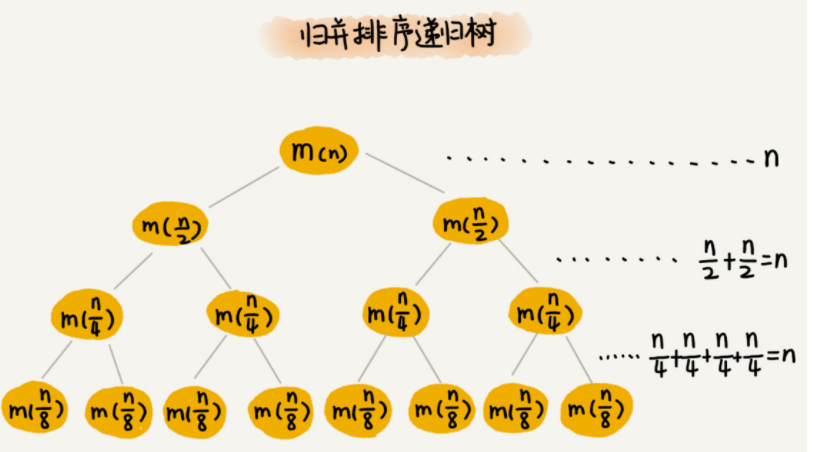因为每次分解都是一分为二，所以代价很低，我们把时间上的消耗记作常量 1。归并算法中比较耗时的是归并操作，也就是把两个子数组合并为大数组。从图中我们可以看出，每一层归并操作消耗的时间总和是一样的，跟要排序的数据规模有关。我们把每一层归并操作消耗的时间记作 n。
现在，我们只需要知道这棵树的高度 h，用高度 h 乘以每一层的时间消耗 n，就可以得到总的时间复杂度 O(n∗h)。
从归并排序的原理和递归树，可以看出来，归并排序递归树是一棵满二叉树。我们前两节中讲到，满二叉树的高度大约是 log2​n，所以，归并排序递归实现的时间复杂度就是 O(nlogn)。我这里的时间复杂度都是估算的，对树的高度的计算也没有那么精确，但是这并不影响复杂度的计算结果。
总结
归并排序的时间复杂读为nlogn,每一行时间复杂度O(n) 然后二叉树高度log(n)
参考
归并排序图解_鸭梨的博客-CSDN博客 
展开全文递归树
• 文章目录1. 基本思想2. 代码实现2.1 递归实现2.2 优化—非递归实现3...归并排序与快速排序的思想基本一致,唯一不同的是归并排序的基准值是数组的中间元素 快排 Link：[排序算法] 6. 快速排序多种递归、非递归实现及性能
•算法 排序
• 排序(Sorting) 是计算机程序设计中的一种重要操作，它的功能是将一个数据元素（或记录）的...本文主要介绍快速排序算法和归并排序算法的基本概念、原理以及具体的实现方法，并对这两种排序算法的时间复杂度进行分析。
• 今天讲讲，为什么归并排序时间复杂度是θ(nlgn) 我们知道归并排序是利用 递归函数 进行计算的一种分治排序算法，因此对其时间复杂度也可以用递归的方式进行计算。 首先我们要知道分治算法的流程： 分解、计算（将...算法
• 归并排序时间复杂度任何情况下都是 O(nlogn)，看起来非常优秀。...那我现在问你，归并排序的空间复杂度到底是多少呢？是 O(n)，还是 O(nlogn)，应该如何分析呢？ 如果我们继续按照分析递归时间数据结构
• 众所周知，归并排序时间复杂度是O(N*lgN) 归并排序时间复杂度推导书上网上一抓一把，但是多数证明都是基于N=2k这个假设来证明的，下面我给出一般情况的证明。 先上归并排序代码： public class MergeSort ...
• 归并排序方法就是把一组n个数的序列，折半分为两...无论每个序列有多少数都是折中分解，所以分解时间是个常数，可以忽略不计。 则：归并排序总时间=子序列排好序时间+合并时间 如果假设一个序列有n个数的排序时间...数据结构 二叉树
• 通过图解分析归并排序流程，以及使用Master公式计算时间复杂度
• 归并排序是建立在归并操作上的一种有效排序算法，该算法是采用分治法的一个典型应用。 这里的分治如何理解？ 比如我们要统计本县城的高考状元，而一个县城中有很多中学，每个中学又有若干个班级，每个班级有若干名...算法 数据结构
• 无论每个序列有多少数都是折中分解，所以分解时间是个常数，可以忽略不计。 则：归并排序总时间=子序列排好序时间+合并时间 1 如果假设一个序列有n个数的排序时间为T(n)，T(n)是一个关于n的函数，随着n的变化而变化...
• 如何计算归并排序时间复杂度？ 什么是归并排序归并排序的概念十分简单，就是“分而治之”的思想。这里我直接从网上找了一份对归并排序算法的比较好的介绍排序算法 。 计算时间复杂度 关键是怎么计算时间复杂度...数据结构 算法
• 在排序算法中快速排序的效率是非常高的，但是还有种排序算法的效率可以与之媲美，那就是归并排序归并排序和快速排序有那么点异曲同工之妙，快速排序：是先把数组粗略的排序成两个子数组，然后递归再粗略分两个子...
• 归并排序 归并排序是分而治之的排序算法。 划分步骤很简单：将当前数组分成两半（如果N是偶数，则将其完全平等，或者如果N是奇数，则一边稍大于一个元素），然后递归地对这两半进行排序。 递归写法 归并排序递归写法...算法 数据结构 java 排序算法
• 算法 时间avg 时间min 时间max 空间avg 稳定性 归并 O(nlog(n)) O(n) O(nlog(n)) n 稳定
• 我们对n个元素进行归并排序，需要时间T(n)， 那分解成两个子数组排序的时间都是T(n/2)。...归并排序时间复杂度计算公式是： T(1) = C； n=1 时，只需要常量级的执行时间，所以表示为 C。 T(n) = 2*T(n/2...
• 归并排序 大体思路 先二分出左右二侧 左侧排好序 右侧排好序 ，申请一个与原数组相同长度的数组，和二个指针分别指向已排好序的左右数组起点位置index = 0 和index=mid+1 此时 如果左右二侧数组为{2,6,9,3,6,8} ...算法 指针
• 前两篇文章中分别是要用递归树、代换法对归并排序时间复杂度进行了简单的分析和证明，经过两次分析后，我们发现递归树法的特点是：可以很直观的反映出整个归并排序算法的各个过程，但因为要画出递归树所以比较麻烦...
• /* * （二分）归并排序(稳定算法) * 基本思想：将数组递归分成越来越小的数集，直到每个数集只有一个数 ...* 空间复杂度分析：归并排序需要一个临时temp[]来储存归并的结果，所以 O(n) * * 基本...java
• 这是《漫谈经典排序算法系列》第四篇，解析了归并排序。  各种排序算法的解析请参考如下： 《漫谈经典排序算法:一、从简单选择排序到堆排序的深度解析》 《漫谈经典排序算法:二、各种插入排序解析及性能...
• 实现归并排序并验证时间复杂度 问题概述 给出一无序数列，要求对其进行排序。那么我们可以使用冒泡排序或者插入排序来实现。然而，当该数列规模较大时，前述两种排序方式的时间复杂度为O(n)=n^2，所以我们需要更加...算法 数据结构
• 引言： 算法是计算机科学中的基础，程序=算法+数据结构，算法描述了我们将如何来处理数据的过程。本文将介绍两种算法的实现以及其中一种算法的复杂度分析过程。算法分析
• 归并排序的递归过程如下，该递归树的高度为log2n（计算过程：假设待排序的数组元素个数为n，设高度为x，x意味着n个元素需要连续二分x次才剩下1个元素，即n/2^x=1，x=log2n），每一层的总比较次数为n，所以时间复杂度......